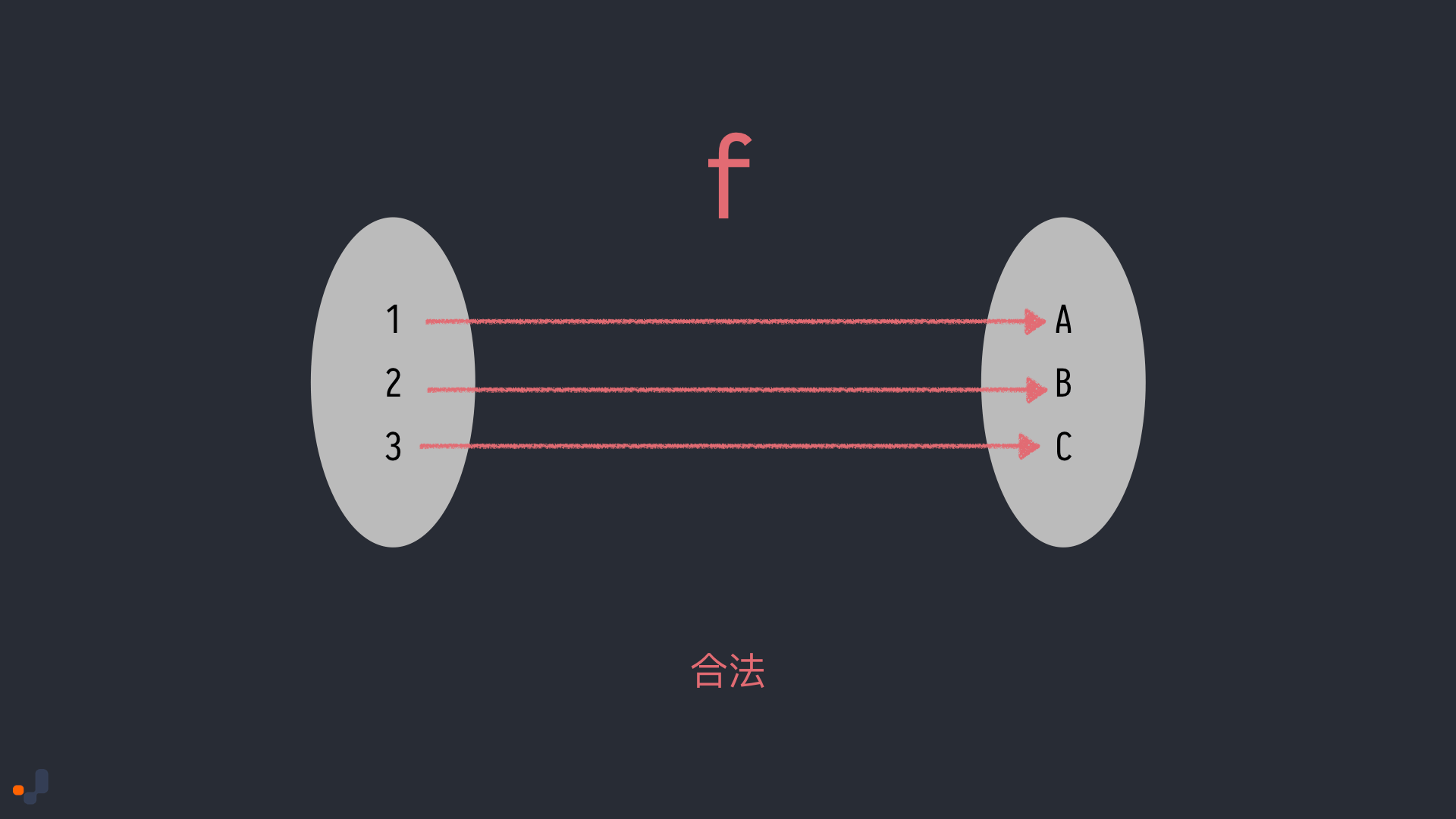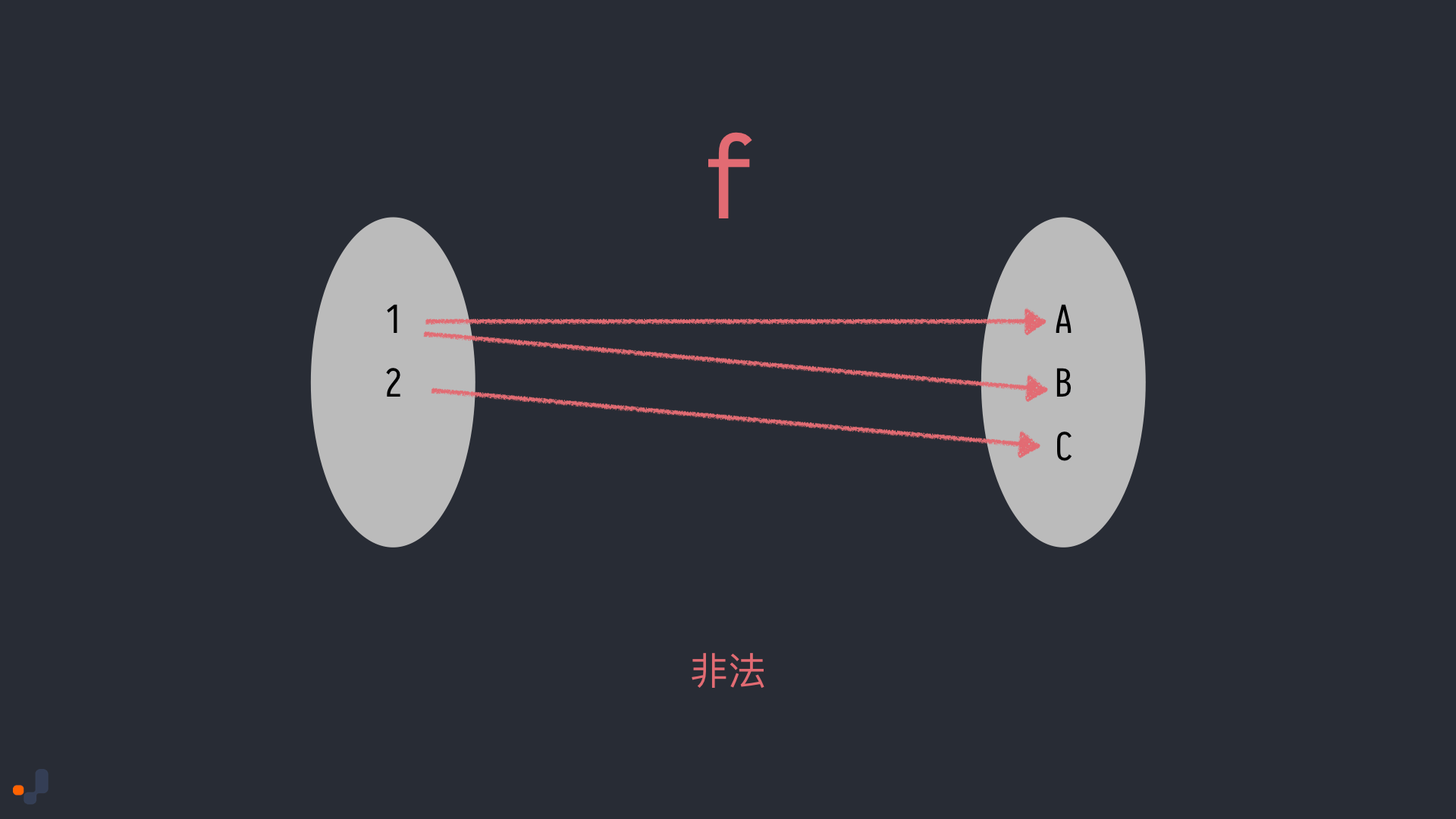# Think in FP (02): All You Need Is Function

Nov 4th, 2019. 4 mins read

## Functional Programming 是什麼?

Functional Programming(FP) 是一種編程範式(programming paradigm)，FP 的核心思想就是透過 function 來解決各種問題，並且所有的 function 都是以「數學函數」為模型。在 Functional Programming 裡 function 是有更明確的定義的：

「functions 是一種表達式，可以輸入參數，一但輸入參數後就可以被簡化(reduced)或是說被運算(evaluated)

add(1)(2) // 可以被簡化成 3

## Function

### 一等公民 (First-Class)

function request(onSuccess) { // onSuccess 是一個 function 被當作參數傳給 request
// ...
// onSuccess(data)
}

return function (y) { // function 被當作 add 的回傳值
return x + y;
}
}

const hello = () => 'hello world!'; // function 當作 value 賦值給 hello 變數

### 引用透明 (Referential Transparency)

const arr = [1, 2, 3];

arr.slice(0, 1) // return 
arr.slice(0, 1) // return 
// slice 是引用透明的，不管執行幾次，只要傳入的參數相同，永遠都會拿到相同的回傳值，
const arr = [1, 2, 3];

arr.splice(0, 1) // return 
arr.splice(0, 1) // return 
// splice 不是引用透明的，splice 改變了 arr 的資料，對外部世界造成了改變

Referential Transparency 的 Function 又可稱為 Pure Function。差別只在於 Pure Function 指的就是 Function 而 Referential Transparency 則可以用在各種 expression 上。 Referential Transparency 也可以理解為，一個 expression 可以直接替換成他的運算結果，並且不對整個程式有任何影響。

## 抽象的來看 Function

$f(1) = A$
$f(2) = B$
$f(3) = C$$f(1) = A$
$f(1) = B$
$f(2) = C$$f(1) = A$
$f(2) = A$
$f(3) = A$Prev

Next